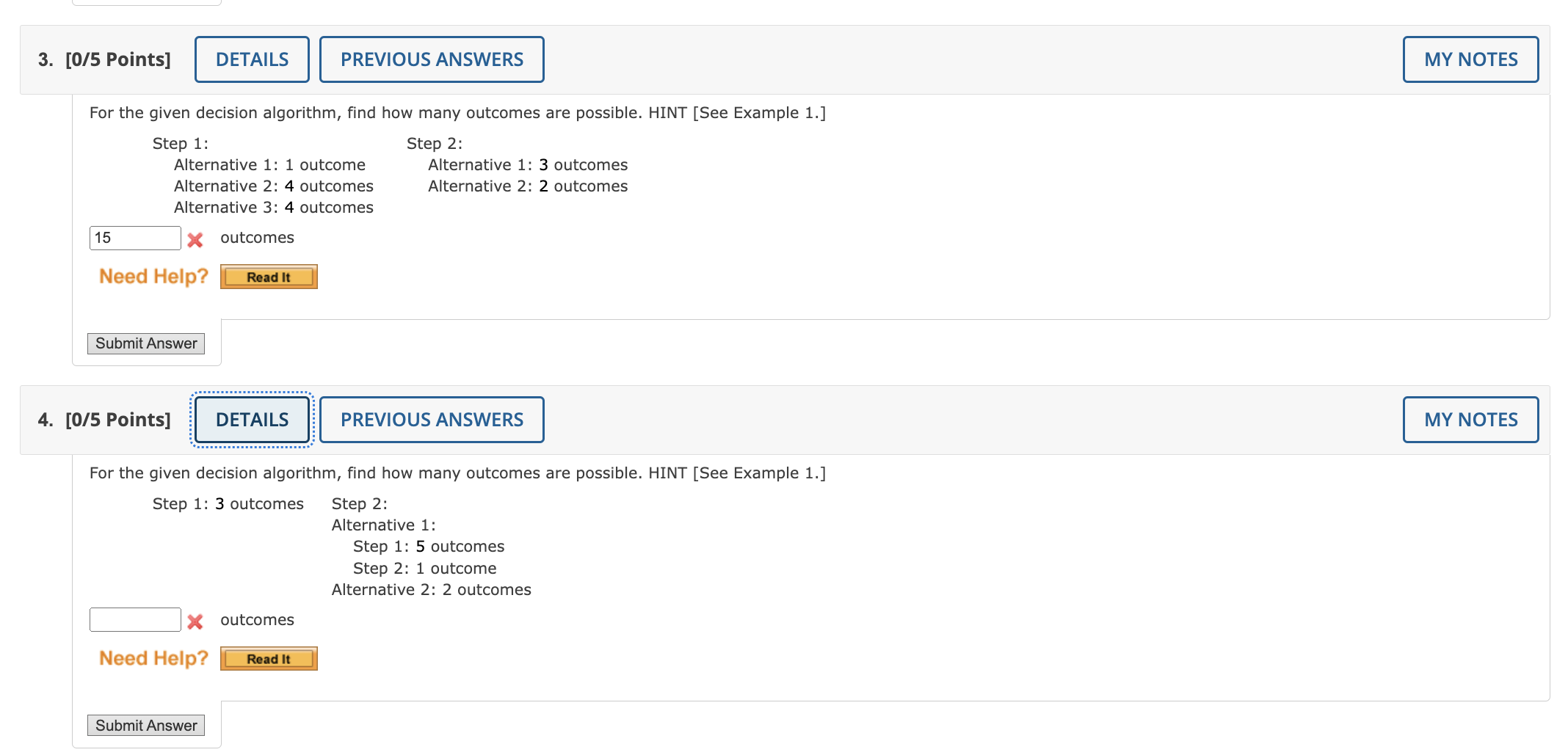Home / Expert Answers / Statistics and Probability / for-the-given-decision-algorithm-find-how-many-outcomes-are-possible-hint-see-example-1-step-1-pa820

# (Solved): For the given decision algorithm, find how many outcomes are possible. HINT [See Example 1.] Step 1 ...For the given decision algorithm, find how many outcomes are possible. HINT [See Example 1.] Step 1: Alternative 1: 1 outcome Alternative 2: 4 outcomes Alternative 3: 4 outcomes outcomes Step 2: Alternative 1: 3 outcomes Alternative 2: 2 outcomes [0/5 Points] For the given decision algorithm, find how many outcomes are possible. HINT [See Example 1.] Step 1: 3 outcomes Step 2: Alternative 1: Step 1: 5 outcomes Step 2: 1 outcome Alternative 2: 2 outcomes outcomes

We have an Answer from Expert